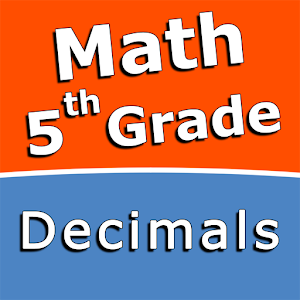English

Every day we offer FREE licensed Android apps and games you’d have to buy otherwise.\$2.49
EXPIRED

Android Giveaway of the Day - Decimals - Fifth grade Math skills

Practice and improve your math skills!
\$2.49 EXPIRED
User rating: 0 (0%) 0 (0%)

This giveaway offer has expired. Decimals - Fifth grade Math skills is now available on the regular basis.

Intuitive interface powered by handwriting input and a choice of three fun and engaging mini games in addition to a regular math trainer mode make our app stand out from the crowd of generic math learning apps.

With Decimals - Fifth grade Math skills you can practice and improve the following math skills:
- Add decimal numbers
- Subtract decimal numbers
- Multiply a decimal by a power of ten
- Multiply a decimal by a one-digit whole number
- Multiply two decimal numbers
- Divide decimals by powers of ten
- Division with decimal quotients
- Divide decimals
- Convert decimals to fractions and mixed numbers
- Convert fractions and mixed numbers to decimals

Choose between four different modes:
- Math Trainer
- Math Shot mini-game
- Math Gravity mini-game
- Math Trick Shot mini-game

Sergey Malugin

Educational

1.0.0

11M

Everyone

4.0 and up

Comments on Decimals - Fifth grade Math skills

Thank you for voting!
Please add a comment explaining the reason behind your vote.
Windows Giveaway of the Day\$29.95
free today
The most advanced screen recording software.